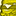# App Development

Announcements
Qlik Cloud Maintenance is scheduled between March 27-30. Visit Qlik Cloud Status page for more details.
cancel
Showing results for
Did you mean:Contributor II

## Sum expression by variable with calculation

I am trying to create a Sum expression with a condition based on a variable minus n.

I have a variable vLatestMonth which has the value of 7.

I am trying to sum the [amount] column where the [month] column is equal to vLatestMonth minus one.

I have tried the following code but received the following error message:

``````SUM({<[month]={\$(vLatestMonth)-1}>}[amount])

Error in set modifier ad hoc element list: ',' or ')' expected``````

Labels (1)
• ### General Question

1 Solution

Accepted SolutionsContributor II
Author

I have found a solution:

``SUM({<[month]={\$(=vLatestMonth-1)}>}[amount])``Contributor II
Author

I have found a solution:

``SUM({<[month]={\$(=vLatestMonth-1)}>}[amount])``Tags
Community Browser